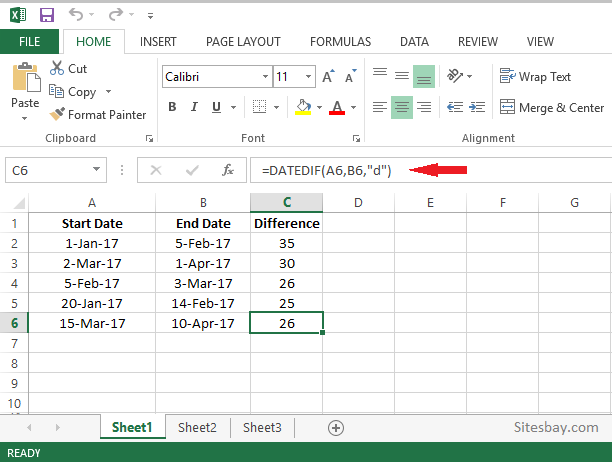# Calculate Difference Between two Dates in Excel

## Calculate Difference Between two Dates in Excel

To Calculate Difference Between two Dates in excel, first make all cell (start date and end date) date type. Here we use predefined formula DATEDIF() and press the Enter key to get the result.

Use the DATEDIF function when you want to calculate the difference between two dates. First put a start date in a cell, and an end date in another. Then type a formula like one of the following.

## Syntax

```DATEDIF(start_date, end_date, unit)
```

## Syntax

```DATEDIF(Start_date,End_date,"d")
```## Syntax

```DATEDIF(Start_date,End_date,"m")
```

## Syntax

```DATEDIF(Start_date,End_date,"y")
```
UnitMeaningExplanation
YYearsNumber of complete years between the start and end dates.
MMonthsNumber of complete months between the dates.
DDaysNumber of days between the start date and end date.
MDDays excluding years and monthsThe date difference in days, ignoring months and years.
YDDays excluding yearsThe date difference in days, ignoring years.
YMMonths excluding days and yearsThe date difference in months, ignoring days and years.

### Calcuate number of days from today

Use TODAY() function to find number of days from today date.

## Syntax

```DATEDIF(TODAY(), "5/20/2015", "d")
```